Example 5.2 Reading From and Creating a Data Set

This example demonstrates how to use the READ DATA statement to read parameters from a SAS data set. The objective is the Bard function, which is the following least squares problem with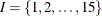: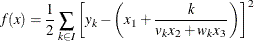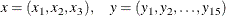where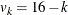,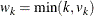(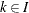), and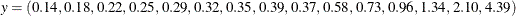The minimum function value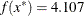E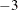is at the point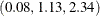. The starting point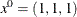is used. This problem is identical to the example Using the DATA= Option in Chapter 7: The NLP Procedure in SAS/OR 12.3 User's Guide: Mathematical Programming Legacy Procedures. The following statements use the READ DATA statement to input parameter values and the CREATE DATA statement to save the solution in a SAS data set:

data bard;
input y @@;
datalines;
.14  .18  .22  .25  .29  .32  .35  .39
.37  .58  .73  .96 1.34 2.10 4.39
;
proc optmodel;
set I = 1..15;
number y{I};
read data bard into [_n_] y;
number v{k in I} = 16 - k;
number w{k in I} = min(k, v[k]);
var x{1..3} init 1;
min f = 0.5*
sum{k in I}
(y[k] - (x + k /
(v[k]*x + w[k]*x)))**2;
solve;
print x;
create data xdata from [i] xd=x;


In these statements the values for parameter y are read from the BARD data set. The set I indexes the terms of the objective in addition to the y array.

The preceding statements define two utility parameters that contain coefficients used in the objective function. These coefficients could have been defined in the expression for the objective, f, but it was convenient to give them names and simplify the objective expression.

The result is shown in Output 5.2.1.

Output 5.2.1: Bard Function Solution

 x
1 0.08241
2 1.13303
3 2.34370

The final CREATE DATA statement saves the solution values determined by the solver into the data set XDATA. The data set contains an observation for each x index. Each observation contains two variables. The output variable i contains the index, while xd contains the value for the indexed entry in the array x. The resulting data can be seen by using the PRINT procedure as follows:

proc print data=xdata;
run;


The output from PROC PRINT is shown in Output 5.2.2.

Output 5.2.2: Output Data Set Contents

Obs i xd
1 1 0.08241
2 2 1.13303
3 3 2.34370